### Geometrical Shapes - Worksheets

CBSE Worksheet-01

CLASS – V – Mathematics – Three Dimensional Figures

Identify the three dimensional figures given below:

 1.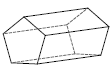2.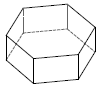3.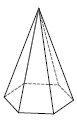4.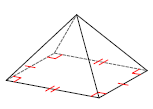5.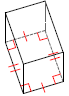6.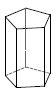7.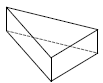8.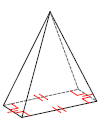9.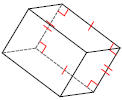10.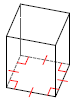CBSE Worksheet-01
CLASS – V – Mathematics – Three Dimensional Figures

1. Pentagonal prism

2. Hexagonal prism

3. Hexagonal pyramid

4. Rectangular pyramid

5. Rectangular prism

6. Pentagonal prism

7. Triangular prism

8. Rectangular pyramid

9. Rectangular prism

10. Square prism